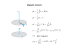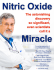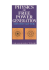# Esters of Nitric Acid as Electron Acceptors

## Transcription

Esters of Nitric Acid as Electron Acceptors
```BULLETIN D E L'ACADÉMIE
POLONAISE DES SCIENCES
S é r i e des sciences c h i m i q u e s
V o l u m e X V I I I , No. 7,
1970
ORGANIC
CHEMISTRY
Esters of Nitric Acid as Electron Acceptors
by
B. H E T N A R S K I , W. POŁUDNIKIEWICZ, and T. URBAŃSKI
Presented by T. URBAŃSKI
on March 2, 1970
It is well known that C-nitro compounds are strong electron acceptors forming
readily charge transfer complexes. Nothing so far has been known as regards possible
electron accepting properties of Onitro compounds. However, in a series of papers
one of us [1—5] and his co-worker  have found using the thermal analysis method
that nitric esters such as D-mannitol hexanitrate and erythritol tetranitrate can
form additive compounds with some aromatic nitro compounds.
The problem arose what is the nature of such additive compounds: whether they
are charge transfer complexes, and which of the components is the electron acceptor.
A suggestion was advanced that nitric esters can act as electron acceptors and
this was substantiated by our present work.
We examined now an interaction between nitric esters of mono-, di-, tri-, tetraand hexahydroxylic alcohols and a model electron donor — tetramethyl-p-phenylenediamine (TMPD). The choice of the latter was justified by its low ionisation
potential (6.5 eV). The preliminary report has already been published .
We found that the solutions of nitric esters, when added to a solution of T M P D ,
produce an intense violet colour. This colour is due to two absorption bands: at
570 and 620 nm (Fig. 1). Their source is the T M P D cation ("Wurster radical")
(I)  formed from T M P D through the loss of one electron.
(I)

В. H e t n a r s k i et-al.
386
620 nm
tOO
500
Я (Vim)
600
Fig. 1. Electronic spectrum of a solution
of glycerol trinitrate (NG) and tetramethyl-p-phenylenediamine ( T M P D ) in 1,2-dichloroethyne at the concentration of 0.018 M of
each component
Fig. 2. Job's diagram in 1,2-dichloroethane;
NG+TMPD,
Erythritol
tetranitrate+TMPD;
/еп-Butyl nitrat e + T M P D . Concentration of each com­
ponent, 0.01 M , thickness, 1 cm. c-conccntration of T M P D in mole fractions
Using Job's method of continuous changes  (Fig. 2) we established that
three O N 0 groups are needed to form one cation (I). Thus, three moles of each
of the nitrates of primary, secondary, and tertiary butyl produced (I) according
to (1):
2
N(CH )
I
3
(1)
3RON0
2
2
N(CH ).
3
+
(3 D O N Q , f K © )
N(CN )
3
2
(II)
Here R denotes primary, secondary, and tertiary butyl, respectively.
Table I gives values of the absorption of T M P D cation which are characterizing
the relative electron affinity of the butyl nitrates.
TABLE I
Electron acceptor,
butyl nitrate
Primary
Secondary
Tertiary
Absorption
at 620 nm *
0.100
0.158
0.530
•Solutions in 1,2-dichloroethane at con­
centration of 0.1 M . The path length was 1 cm.
It follows from the above data that the primary O N 0 group creates the weakest
electron acceptor properties of butyl nitrates, and the strongest were shown by the
2
Esters of Nitric Acid as Electron
Acceptors
387
tertiary 0 N 0 group. This is most likely the result of the strongest electron delocalization when O N 0 is attached to the branched aliphatic group.
2
2
Ethylene glycol dinitrate reacted with T M P D at the same ratio of components:
three moles of the dinitrate with one mole of T M P D .
Glycerol trinitrate (nitroglycerine, NG), reacted with T M P D at the molar ratio
1:1, i.e. three O N 0 groups were needed to form (I). Its absorption produced by
the cation T M P D at 620 nm is 0.272 (concentration of the components was
0.018 mol/1., the layer thickness, 1cm).
2
Pentaerythritol trinitrate shows the same electron afinity as N G , the molar
ratio being 1:1.
Two tetranitrates were also examined: erythritol tetranitrate and pentaerythritol
tetranitrate (PETN). Both react with T M P D and the molar ratio of tetranitrate:
T M P D was found to be 1:1. Erythritol tetranitrate is more reactive, i.e. has stronger
electron acceptor properties (Table П).
T A B L E II
Absorption
at 620 nm *
Concentration,
M
PETN
0.100
0.02
Erythritol tetranitrate
0.540
0.01
Electron acceptor
* The path length was 1 cm.
It seems that the higher electron acceptor properties of erythritol tetranitrate
to form complexes can partly be explained by a more polar structure of that ester,
as suggested in one of the former papers .
T A B L E III
Electron acceptor
Absorption
at 620 nm *
D-Mannitol hexanitrate
Dulcitol hexanitrate
D-Sorbitol hexanitrate
игио-Inositol hexanitrate
* Concentration of components was
path length, 0.02 cm.
0.499
0.455
0.468
0.530
2xlO
- 3
M,
Nitric esters of a number of hexahydroxylic alcohols were also examined: viz.
hexanitrates of D-mannitol, dulcitol (D- or L-galactitol), D-sorbitol and mj>o-inositol.
Using the same method of continuous changes we found that one mole of hexanitrates
reacted with two moles T M P D , i.e. the rule of three O N 0 groups for one mole
2
В. H e t n a r s k i et al.
388
of T M P D is fulfilled. The electron affinity of the hexanitrates is much stronger
than that of nitric esters with a lower number of O N 0 groups (Table III).
The reaction of hexanitrates with T M P D was manifested not only by the for­
mation of the Wurster radical. The latter was subjected to disproportionation yielding
a divalent cation of tetramethyl-/>benzoquinone-diimonium which in turn formed
a charge transfer complex. This will be the object of our next paper .
2
Conclusions
On the basis of our experiments we can formulate a general rule for the relation
between the number of O-nitro groups in nitric esters of alcohols, the number of
moles of the ester and number of moles of T M P D , according to the schematic
equation:
x R ( O N 0 ) „ + / r M T P ^ [xR(ON0 ),,] ' y [ T M P D ]
J a
2
3
2
where R are hydrocarbon groups.
Table IV gives the values of x and y as the function of n.
T A B L E IV
Values of
11
X
У
1 or 2
3
1
3 or 4
1
1
6
1
2
The general rule reads: at least three O-nitro groups should be present to form the
It should also be pointed out that inorganic nitrates such as potassium, sodium
and aluminium nitrates do not react with T M P D . The observed electron affinity
of nitrates is limited to nitric esters only and should be ascribed to délocalisation
of electrons along the bonds С—O—N0 .
2
Experimental
1 , 2 - D i c h l o r o e t h a n e (as solvent) was purified according to the literature [13J.
T c t r a m e t h y l - p - p h e n y l c n c d i a m i n e was prepared according to the literature  and
purified by double distillation in the atmosphere of nitrogen (m.p. 51°).
л - B u t y l a n d s e c - b u t y l n i t r a t e s . The corresponding butyl alcohol was carefully added
at —10° under vigorous stirring to an excess of nitrating mixture of H N 0 (dl.5) and H S 0
Ul 1.84) (1:1 wt./wt.). Subsequently all was poured into water and ice, extracted with ether, washed
with aq. sodium carbonate, dried over sodium sulphate and the product was distilled under re­
duced pressure ( l O m m H g ) .
/ e r r - B u t y l n i t r a t e was prepared by mixing cooled tor-butyl chloride in dry ether with
silver nitrate in the molar ratio 1:3. A l l was left in a refrigerator for two days, the solid phase was.
3
2
4
Esters
of Nitric Acid as Electron
Acceptors
389
filtered off, ether solution was washed first with water, then with 5% solutions of sodium carbo­
nate and sodium hydrogen sulphite, and dried over sodium sulphate. The product was distilled
under reduced pressure (5 mm Hg).
E t h y l e n e g l y c o l d i n i t r a t e and g l y c e r o l t r i n i t r a t e were prepared by standard methods
 and purified by distillation under reduced pressure (20 mm Hg) and by freezing crystallization
, respectively.
P e n t a e r y t h r i t o l t r i n i t r a t e was prepared and purified according to the literature .
E r y t h r i t o l a n d p e n t a e r y t h r i t o l t e t r a n i t r a t e s were prepared according to the litera­
ture [17, 14]. The products were purified by crystallization from E t O H .
TABLE V
20
I'D
Substance
«-Butyl trinitrate
sec-Butyl trinitrate
tert-Butyl nitrate
Ethylene glycol dinitrate
Glycol trinitrate
Pentaerythritol trinitrate
Erythritol tetranitrate
Pentaerythritol tetranitrate
D-Mannitol hexanitrate
Dulcitol hexanitrate
D-Sorbitol hexanitrate
ш.г-0-Inositol hexanitrate
m.p.
b.p.
-
25°/8 mm
22°/8 mm
22°/5 mm
105720 mm
—
-
-
61°
141°
112°
9 4 - 94.5°
5 4 - 54.5°
132-132.5°
—
1.4063
1.4025
1.4020
1.4475
1.4732
1.4936
—
—
—
—
—
—
—
Hexanitrates of D-mannitol, dulcitol, D-sorbitol, />i.yo-inositol were prepared according to the
literature [15, 19].
The properties of the substances are collected in Table V .
The electronic absorption spectra were taken on a Unicam SP 500 spectrophotometer.
The authors are indebted to D r R. Kuboszek and M r S. Krzemiński, M . Sc., for the prepa­
ration of ethylene glycol dinitrate, glycerol and pentaerythritol trinitrates.
INSTITUTE O F O R G A N I C C H E M I S T R Y , POLISH A C A D E M Y O F SCIENCES, WARSAW,
KASPRZA­
K A 48/52
(INSTYTUT CHEMII ORGANICZNEJ P A N , WARSZAWA)
D E P A R T M E N T O F C H E M I S T R Y , T E C H N I C A L U N I V E R S I T Y , W A R S A W , K O S Z Y K O W A 75
( K A T E D R A T E C H N O L O G I I O R G A N I C Z N E J II P O L I T E C H N I K I , W A R S Z A W A )
REFERENCES
[I] T. U r b a ń s k i , Roczniki Chem., 13 (1933), 399.

, ibid., 14 (1934), 925.
[31
, ibid., 15 (1935), 191.

, ibid., 16 (1936), 359.

, ibid., 17 (1937), 474.
 M . W i t a n o w s k i , ibid., 39 (1965), 635.
17] B. H e t n a r s k i , W . P o ł u d n i k i e w i c z , T. U r b a ń s k i , Tetrahedron Lett., 1970, 3.
390
В . H e t n a r s k i et al.
 G . B r i e g l e b , Elektionen-Donator-Acceptor-Komplexe, Springer, Berlin, 1961.
 A . C . A l b r e c h t , W . T. S i m p s o n , J. A m . Chem. S o c , 77 (1955), 4454.
 P. J o b , Compt. Rend., 180 (1925), 928; A n n . Chim. Phys., (10) 9 (1928), 113.
 T. U r b a ń s k i , Roczniki Chem.. 25 (1961), 257.
 T. U r b a ń s k i , В. H e t n a r s k i , W . P o t u d n i k i e w i c z , Bull. Acad. Polon. Sci.. Sér. Sci.
Chim., [sec the following paper in this issue].
 J. C . D. B a n d , Snedder, Trans. Faraday S o c , 53 (1957), 894.
 J. N . A s h l e y , W . G . Leeds, J. Chem. S o c , (1957), 2706.
 T. U r b a ń s k i , Chemistry and technology of explosives, Vol. II, Pergamon Press, Oxford —
P W N , Warszawa, 1965.
 J. H a c k e l , Roczniki Chem., 16 (1936), 213.
 N . S. M a r a n s , D. E . E l r i c k , and R . T. P r e c k e l , J. A m . Chem. S o c , 76 (1954), 1304.
 A . S a s k i s y a n t s , Med. Prom. SSSR, 14 (1960), 117.
 T. U r b a ń s k i , M . W i t a n o w s k i , Trans. Farad. S o c , 59 (1963), 1039.
```

### ELECTRON ENERGY LOSS SPECTROSCOPY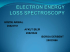### What is the Electron Transport Chain?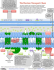### Folie 1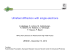### Electron microscopy has played a major role in the understanding of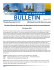### Chapter 12 The Periodic Table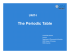### Adobe Acrobat file () - Wayne State University Physics and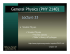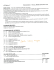### Resonance Structures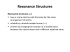### Lecture 14: Nuclear Fusion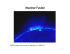### Periodic Properties of the Elements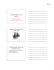### rutherfords model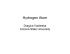### J.S. Yoon (NFRI) - IAEA Atomic and Molecular Data Unit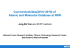### Page 345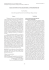### Correlative Light and Electron Microscopy using Immunolabelled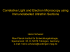### bionapl/3d - Science - University of Waterloo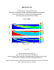### spdfgh